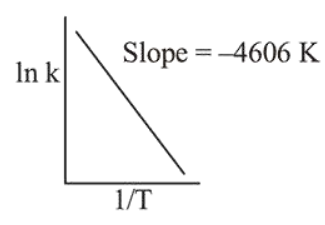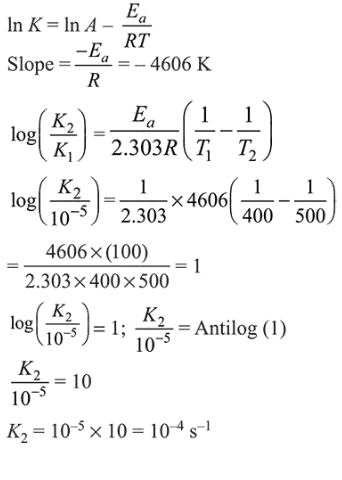# For a reaction, consider the plot ofQuestion:

For a reaction, consider the plot of $\ln \mathrm{k}$ versus $1 / \mathrm{T}$ given in the figure. If the rate constant of this reaction at $400 \mathrm{~K}$ is $10^{-5} \mathrm{~s}^{-1}$, then the rate constant at $500 \mathrm{~K}$ is :1. For a reaction, consider the plot of

2. $2 \times 10^{-4} \mathrm{~s}^{-1}$

3. $10^{-4} \mathrm{~s}^{-1}$

4. $4 \times 10^{-4} \mathrm{~s}^{-2}$

Correct Option: , 3

Solution:

From Arrhenius equation,